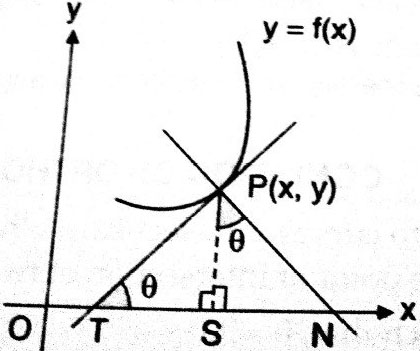# The length of tangent drawn at (4, 4) on the curve  is ?  Option 1) Option 2) Option 3) Option 4)

H Himanshu

As we have learned

Length of Tangent -

- whereinWhere

length of tangent

at (4,4) is 1/2

length =

Option 1)

Option 2)

Option 3)

Option 4)

Exams
Articles
Questions